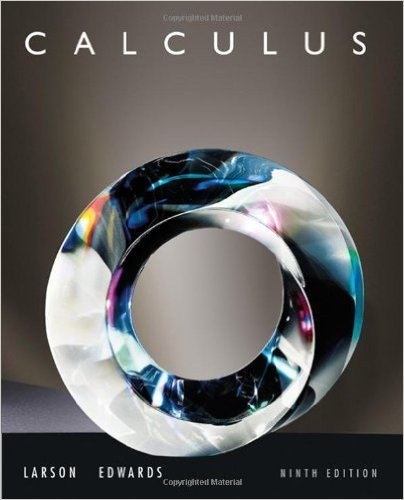×
×

# Solutions for Chapter 15.4: Greens Theorem## Full solutions for Calculus | 9th Edition

ISBN: 9780547167022Solutions for Chapter 15.4: Greens Theorem

Solutions for Chapter 15.4
4 5 0 287 Reviews
10
0
##### ISBN: 9780547167022

This textbook survival guide was created for the textbook: Calculus , edition: 9. This expansive textbook survival guide covers the following chapters and their solutions. Chapter 15.4: Greens Theorem includes 50 full step-by-step solutions. Since 50 problems in chapter 15.4: Greens Theorem have been answered, more than 63007 students have viewed full step-by-step solutions from this chapter. Calculus was written by and is associated to the ISBN: 9780547167022.

Key Calculus Terms and definitions covered in this textbook
• Algebraic expression

A combination of variables and constants involving addition, subtraction, multiplication, division, powers, and roots

• Algebraic model

An equation that relates variable quantities associated with phenomena being studied

• Blind experiment

An experiment in which subjects do not know if they have been given an active treatment or a placebo

• Combination

An arrangement of elements of a set, in which order is not important

• Conic section (or conic)

A curve obtained by intersecting a double-napped right circular cone with a plane

• Demand curve

p = g(x), where x represents demand and p represents price

• Dependent event

An event whose probability depends on another event already occurring

• Empty set

A set with no elements

• Fitting a line or curve to data

Finding a line or curve that comes close to passing through all the points in a scatter plot.

• Focal axis

The line through the focus and perpendicular to the directrix of a conic.

• Frequency table (in statistics)

A table showing frequencies.

• LRAM

A Riemann sum approximation of the area under a curve ƒ(x) from x = a to x = b using x1 as the left-hand endpoint of each subinterval

• n factorial

For any positive integer n, n factorial is n! = n.(n - 1) . (n - 2) .... .3.2.1; zero factorial is 0! = 1

• Normal distribution

A distribution of data shaped like the normal curve.

The measure of an angle in radians, or, for a central angle, the ratio of the length of the intercepted arc tothe radius of the circle.

• Random behavior

Behavior that is determined only by the laws of probability.

• Real number

Any number that can be written as a decimal.

• Reference triangle

For an angle ? in standard position, a reference triangle is a triangle formed by the terminal side of angle ?, the x-axis, and a perpendicular dropped from a point on the terminal side to the x-axis. The angle in a reference triangle at the origin is the reference angle

• Speed

The magnitude of the velocity vector, given by distance/time.

• Zero factor property

If ab = 0 , then either a = 0 or b = 0.

×